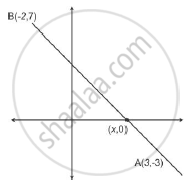# Find the ratio in which the line segment joining the points A(3,- 3) and B(- 2, 7) is divided by x-axis. Also find the coordinates of the point of division. - Mathematics

Find the ratio in which the line segment joining the points A(3,- 3) and B(- 2, 7) is divided by x-axis. Also find the coordinates of the point of division.

#### Solution

Solution:
Point p lies on x axis so it’s ordinate is 0 (Using section formula)Let the ratio be k: 1 Let the coordinate of the point be P(x , 0) As given A(3,-3) and B(-2,7)

The co-ordinates of the point P(x,y), which divide the line segment joining the points A(x1,y1) and B(x2,y2), internally in the ratio m1:m2 are

P_x=(mx_2+nx_1)/(m+n)

P_y=(my_2+ny_1)/(m+n)

Hence, A(3,-3) be the co-ordinates (x1,y1) and B(-2,7) be the co-ordinates (x2,y2)
m = k
n = 1

0 = k x 7 + 1 x -3 / (k+1)
0(k+1) =7k -3
0 =7k - 3
3=7k
k = 3 /7
k:1 = 3 : 7

P_x=(mx_2+nx_1)/(m+n)

P_x=[(3/7xx-2)+(1xx3)]/(3/7+1)=2.41

Concept: Division of a Line Segment
Is there an error in this question or solution?
2013-2014 (March) All India Set 2

Share